# Linear Momentum and Principle of Conservation of Momentum

## Linear Momentum

Momentum is the motion contained in a body. Quantity of motion possessed by a body depends upon both of its mass and velocity. So the product of mass and velocity is the measure of the momentum,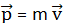It is also called linear momentum. It is a vector quantity whose direction is in the direction of the velocity. Its unit is kg m/s is SI-units and dimension, [M L T-1].

When there is change in the momentum of a given body, there is change in its velocity, i.e. there is acceleration. According to Newton’s first law of motion, the change in motion is produced by external force. So there is a relation between the change in momentum and the force.

## Principle of Conservation of Linear Momentum

The principle of conservation of linear momentum states that,

If no external forces act on the system of two colliding objects, then the vector sum of the linear momentum of each body remains constant and is not affected by their mutual interaction.

Let us consider an isolated system of n particles having initial momentum p1, p2 … pn. Due to the collision, let the momentum of the particles after collision be p1‘, p2 … pn‘ respectively. Then according to the principle of conservation of linear momentum, in the absence of external force,

`p1 + p2 + ... + pn = p1' + p2' + ... + pn'`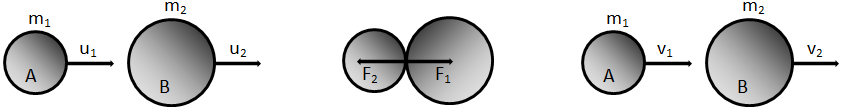Fig: Collision between two spheres moving in same direction in a straight line.

To prove this principle, we consider a collision between two spheres A and B having masses of m1 and m2 respectively. Let u1 and u2 be the velocities of the spheres before collision such that u1 > u2 and moving on the same straight line as shown in the figure. After collision, let their velocities be v1 and v2 on the same line. If they collide with each other for a short time interval t, each sphere exerts a force on the other sphere and so, the force experienced by A is given as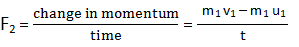Similarly, force experienced by B is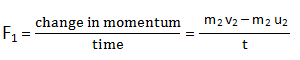According to Newton’s third law of motion, the force experienced by A and B are equal and opposite,

or,

That is, total momentum before collision is equal to total momentum after collision if no external forces act on them which proves the principle of conservation of linear momentum.

### Example: Principle of Conservation of Linear Momentum

Calculate the recoil velocity of a 3.0 kg pistol which shoots a 0.060 kg bullet at a speed of 300 m s-1. (Assume that the total momentum of the system was 0 before bullet was shoot).

The momentum of pistol and bullet should be equal and opposite according to the principle of conservation of linear momentum, i.e,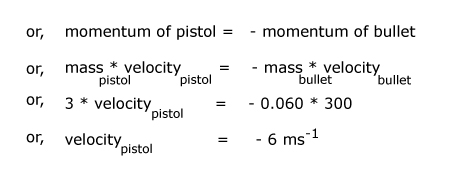The negative sign indicates that, the direction of pistol is opposite to the direction of bullet.